# Problems of the perimeter of a rectangle

#### Number of problems found: 128

• 144 stepsTo get around the garden we have to take 144 steps. 8 steps less in width than in length. One step is 5 decimetres long. What are the dimensions of the garden? What is its perimeter and its area?
• Dimensions 3A perimeter of a rectangular field is 96 meters. The length is four meters less than three times the width. Find the length and width (its dimensions).
• Matilda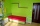Matilda measures the perimeter of her bedroom to determine how many feet of border she needs to go around the tops of the walls. Two walls are each 96 inches long, and two walls are each 3 yards long. She pays \$30 for border that costs \$1 per foot. What a
• Perimeter - rectangleFind the perimeter of the rectangle. A rectangle with a base of (3 5/6) m and a height of (2 3/7) m Its perimeter is x= meters. (Use fraction in your answer. )
• A rectangular 4A rectangular garden has a length of 57 m and a width of 42 m. Calculate of how many m2 will decrease the area of a garden, if the ornamental fence with a width of 60 cm will be planted inside its perimeter.
• Length 9Length of a rectangle is 8 m less than twice of breadth. If the perimeter of the rectangle is 50. find its length and breadth.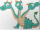Three sides of a quadrilateral are equal to the foreside which is 16 cm long. What is the length of the one with equal sides if the perimeter is 58 cm?
• Dimensions of rectangleFind the dimensions of a rectangle given that its perimeter is 110 cm and its length is 1 cm more than twice its width.
• What is 8What is the perimeter of a rectangle with side lengths of ¾ inch and ⅔?
• Area and perimeter 2Find the area and the perimeter of a rectangle of length 45 1/2 cm and breadth 16 2/3 cm.
• The length 4The length of a rectangular room measures twice a number x increased by 5. The width measures a number x increased by 3. What is the perimeter of the room?
• The homeowner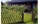The homeowner plans to build a fence along the sides and back of the property. How many feet of fencing are required to build the fence? (1 metre = 3.2808399 feet)Lots: 14w x 36.6d (m)
• Triangular landJana has a rectangular garden measuring 30 meters by 72 meters that she wants to split diagonally from corner to corner using a fence. How long does her fence need to be?
• Angle of diagonalsCalculate the perimeter and the area of a rectangle if its diagonal is 14 cm and the diagonals form an angle of 130°.
• Side and diagonalFind the circumference and the area of the rectangle if given: side a = 8 cm diagonal u = 10 cm.
• A rectangle 4A rectangle has area 300 and perimeter 80. what is the ratio of the length and width?
• Perimeter of a rectangleFind the perimeter of a rectangle with a length of 1/2 feet and a width of 1 1/3 feet
• Harry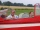Harry Thomson bought a large land in the shape of a rectangle with a circumference of 90 meters. He divided it into three rectangular plots. The shorter side has all three plots of equal length, their longer sides are three consecutive natural numbers. Fi
• Orange and tangerine treesBrad's father asked an engineer to survey the field behind their house. He wanted to plant some orange and tangerine trees there. According to the survey, the field is thirty-two feet long and six yards wide. Questions: What is the area of the field in sq
• A rectangle's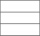A rectangle's length is 3 times more than twice its width. If the perimeter of the rectangle is 60 feet. What is the length of the rectangle

Do you have an exciting math question or word problem that you can't solve? Ask a question or post a math problem, and we can try to solve it.

We will send a solution to your e-mail address. Solved examples are also published here. Please enter the e-mail correctly and check whether you don't have a full mailbox.

Perimeter - math problems. Rectangle Problems.